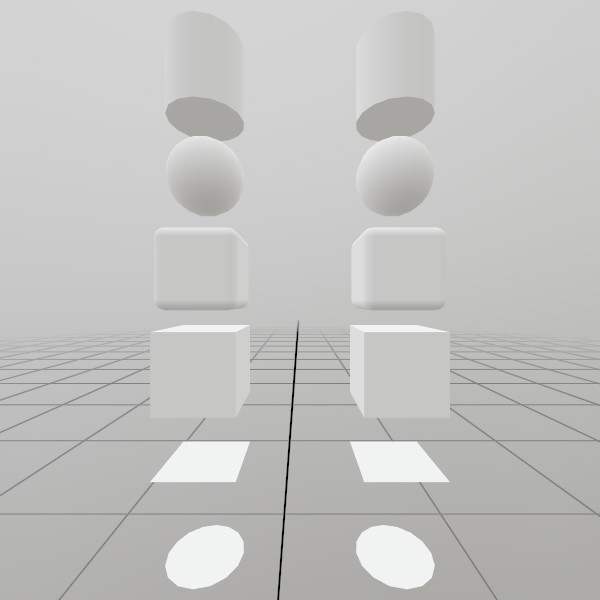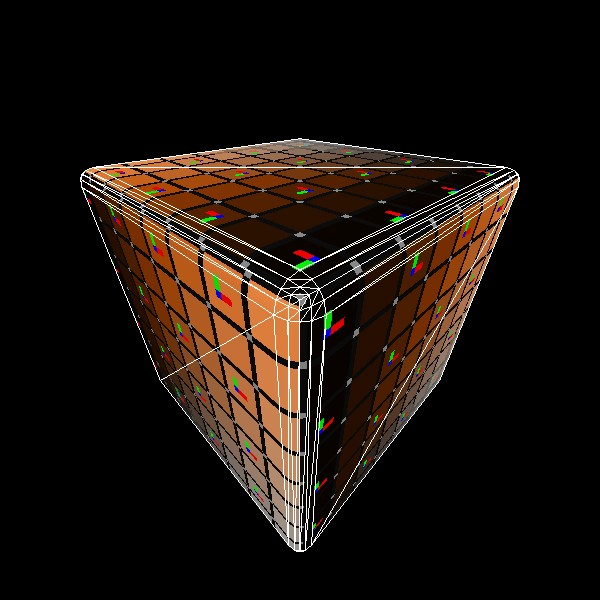# Mesh.GenerateRoundedCube

``````static Mesh GenerateRoundedCube(Vec3 dimensions, float edgeRadius, int subdivisions)
``````

Generates a cube mesh with rounded corners, pre-sized to the given dimensions. UV coordinates are 0,0 -> 1,1 on each face, meeting at the middle of the rounded corners.

NOTE: This generates a completely new Mesh asset on the GPU, and is best done during ‘initialization’ of your app/scene.

Vec3 dimensions How large is this cube on each axis, in meters?
int subdivisions How many subdivisions should be used for creating the corners? A larger value results in smoother corners, but can decrease performance.
RETURNS: Mesh A cube mesh with rounded corners, pre-sized to the given dimensions.

## Examples

### Generating a Mesh and ModelHere’s a quick example of generating a mesh! You can store it in just a Mesh, or you can attach it to a Model for easier rendering later on.

``````// Do this in your initialization
Mesh  roundedCubeMesh  = Mesh.GenerateRoundedCube(Vec3.One * 0.4f, 0.05f);
Model roundedCubeModel = Model.FromMesh(roundedCubeMesh, Default.Material);
``````

Drawing both a Mesh and a Model generated this way is reasonably simple, here’s a short example! For the Mesh, you’ll need to create your own material, we just loaded up the default Material here.

``````// Call this code every Step

Matrix roundedCubeTransform = Matrix.T(-.5f, 0, 0);
roundedCubeMesh.Draw(Default.Material, roundedCubeTransform);

roundedCubeTransform = Matrix.T(.5f, 0, 0);
roundedCubeModel.Draw(roundedCubeTransform);
``````

### UV and Face layout

Here’s a test image that illustrates how this mesh’s geometry is laid out.``````meshRoundedCube = Mesh.GenerateRoundedCube(Vec3.One, 0.05f);
``````

Found an issue with these docs, or have some additional questions? Create an Issue on Github!